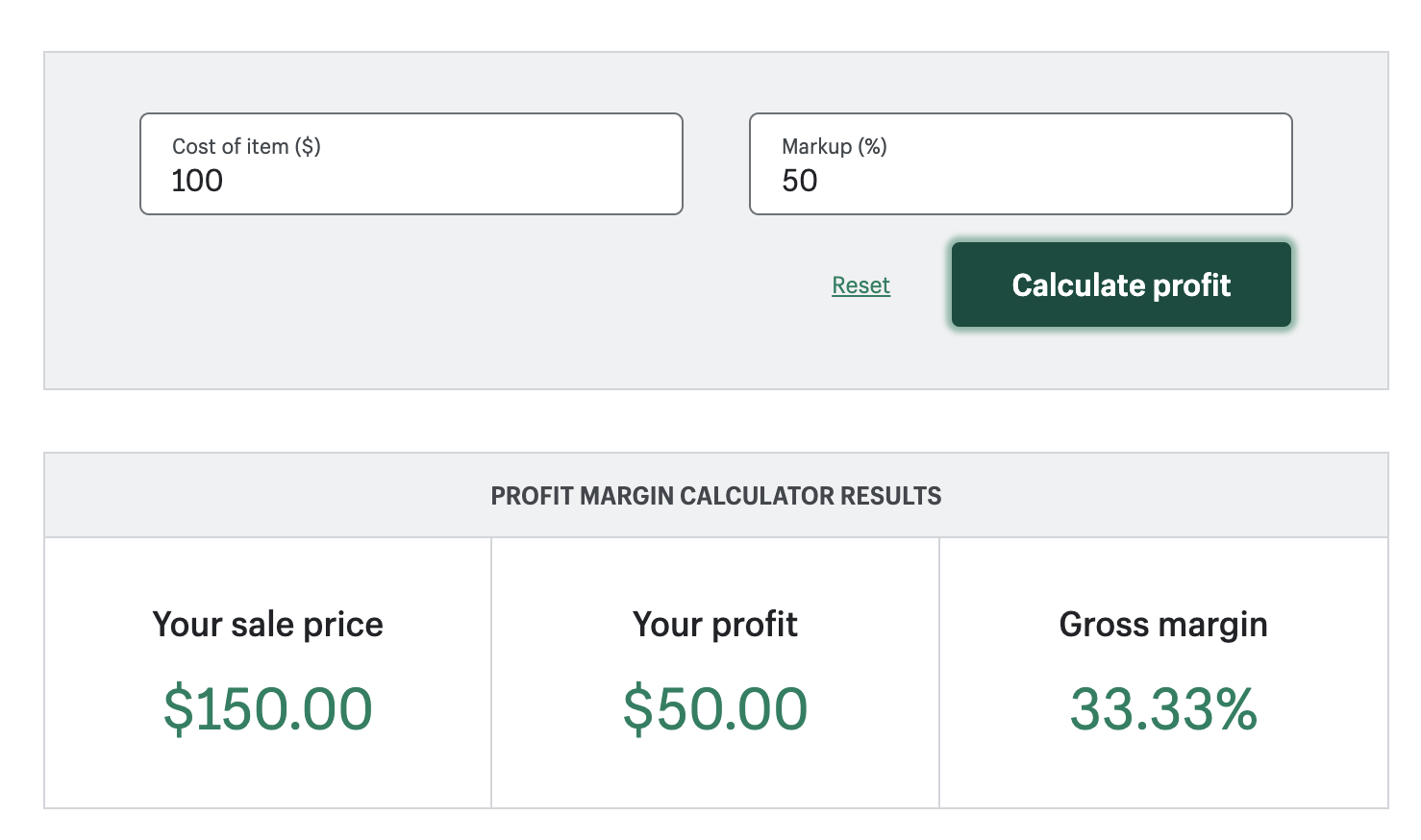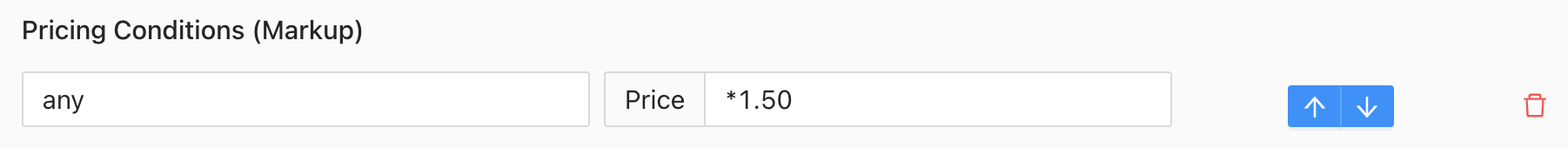What are Markup and Margin?

They are percentages that are used to calculate the margins.

Markup is used to calculate the profit rate with Cost as the denominator.
Markup = [Profit / Cost ] x 100

Margin is used to calculate the profit rate with Sales as the denominator.
Margin = [Profit  / Sales ] x 100

How to calculate markup based on profit margin?

1. This is how you calculate markup based on the profit margin by simply using the profit margin calculator

2. From the calculator, you can determine the profit just by entering the cost price and markup percentage.

3. Once you get the result, you just need to take the (Profit / Margin) = Markup Price

For example, Your markup will be (Profit/Margin) = 50/33.33 = 1.50Pricing condition in Stock Sync

Please calculate the markup based on the above steps and put it in the pricing conditions in Stock Sync.

If  50%,

Then use 1.50 to markup the price.For example,This shoe's price is \$100 and you would like to have 50% profit from the selling price. You need to add the markup as this in the pricing condition. \$100 * 1.50 = 150 The profit will be \$50